# I need to find current and voltage on resistor using Thévenin's theorem

#### DrewTheSaint

Joined Oct 21, 2016
1
I'm not really sure how to begin, since our teacher was not able to explain the use of Thévenin's theorem sufficiently (at least for me). What I know so far is, that I am supposed to simplify circuit on terminals where unknown voltage and current is located. But since there are two pairs of terminals, I'm not really sure if I'm supposed to work with both. Can you please get me started?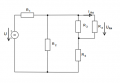We know value of voltage U and resistances of all resistors. We want to calculate IR4 along with UR4.

Thank you.

#### DGElder

Joined Apr 3, 2016
351
"I'm not really sure if I'm supposed to work with both."

Both? I only see one pair of relevant terminals. You answered your own question:

"I am supposed to simplify circuit on terminals where unknown voltage and current is located."...
...."We know value of voltage U and resistances of all resistors. We want to calculate IR4 along with UR4."

Remove the load from those terminals and find the voltage across those terminals to get Vth. Then short the voltage source and find the resistance looking into those terminals to get Rth. Redraw the circuit as the Thevenin equivalent with the load reattached and find the unknown current and voltage.

Last edited:

#### DGElder

Joined Apr 3, 2016
351
Upon review I do see some perceivable ambiguity in the problem statement. That may be because of the original statement or your interpretation, i.e. is the load R3 and R4 or just R4. The most efficacious approach to find the unknown current is to treat R4 as the load; that is what I would do anyway. It doesn't really matter as long as you show your work, make clear what you are considering the load and demonstrate that you know how to use Thevenin theorem to simplify the circuit and get your answer.
.

Last edited:

#### WBahn

Joined Mar 31, 2012
26,398
Although there are many ways to go about obtaining an answer, for this question by far the most reasonable approach is to treat R4 as the load since you want to know the current through R4 and the voltage across R4. That's what the core of using a Thevenin equivalent is all about -- finding the voltage/current relationship at some partition of a linear circuit. So you need to choose a partition that exposes the voltage and current of interest. The ONLY partition of this circuit that does that is to treat R4 as the load.

@DrewTheSaint : Make your best attempt to solve the problem, going as far as you can. It doesn't have to be complete or correct, but it needs to exist in order to give us a starting point.

#### RBR1317

Joined Nov 13, 2010
690
When asked to find the voltage and current through a load resistor (R4) using Thevenin analysis, it means to find the Thevenin equivalent of the driving circuit as seen from the blue terminals in the diagram below. Then use this simplified Thevenin circuit to drive the load resistor.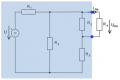In order to get the Thevenin equivalent, one needs to find the open-circuit voltage (Voc) and the short-circuit current (Isc). Then the Thevenin voltage becomes the open-circuit voltage, Vth=Voc, and the Thevenin resistance is calculated, Rth=Voc/Isc.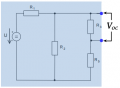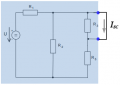Alternately, one can find the Thevenin resistance as the equivalent resistance of the driving circuit (shaded blue) with the sources zeroed, i.e. voltage sources shorted & current sources opened.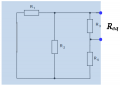•anhnha and DrewTheSaint